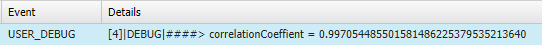Excel CORREL function in Apex Salesforce

Today we will see salesforce apex equivalent code for Excel CORREL function. Excel CORREL function in Apex Salesforce can be used if we need to perform same calculation on salesforce data as excel CORREL  function.

Syntax of CORREL function is as follows in Excel

• CORREL(array1, array2)
• WHERE array1 and array2 both are Required.
• We must ensure that array1 and array2 are same in size.

See the code below, code has static methods written you can put them in your util class/helper class.

Once we have above methods in our code, we can use it 🙂 , Now lets test the method from developer console, open developer console and write below code.

When you execute the above code, you will get the output as follows.correlationCoeffient = 0.997054485501581486225379535213640